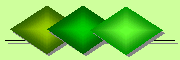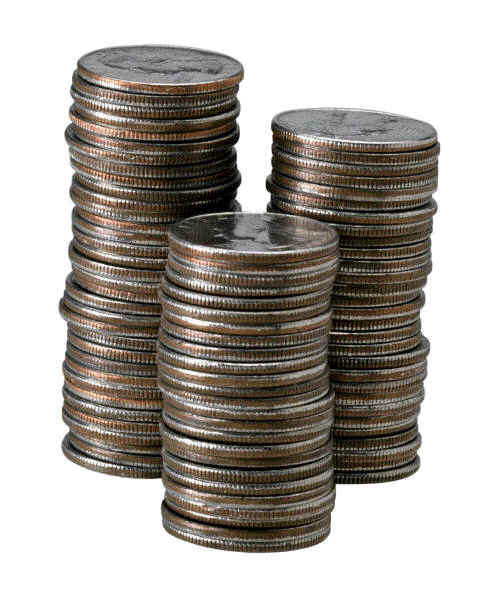De Claron LtdExpress Accountancy services at affordable prices
 Accounting Property Investment Reporting Company Secretarial ServicesHelp

Gross Rent Multiplier

Gross Rent Multiplier (GRM) is the ratio of the or market value of a property in relation to its annual gross income.

• GRM = Market Value / Annual Gross Income
• Annual gross income can be calculated using Gross Potential Income (GPI) or Gross Operating Income (GOI). Though calculations using GPI are easier, GRM calculations using GOI are more accurate as it takes into account losses arising from Vacancy and Credit Loss.

GRM based on GPI is calculated as follows:

• \$100,000 / \$10,000 = 10%
• where:

• \$100,000 = market value of the property
• \$10,000 = GPI
• 10% = GRM
• The GRM above is based on the assumption that all rental units are occupied and that all rent is paid. However, from experience, it has been found that there are annual losses arising from vacancies and non-collections of rent (i.e. from Vacancy and Credit Loss) which average 10% of the total GPI. Given the comparatively high percentage of Vacancy and Credit Loss it is better to base the GRM on GOI.

To calculate GOI merely multiply the GPI figure given above by the percentage of Vacancy and Credit Loss

• \$10,000 x 10% = \$1,000
• \$10,000 - \$1,000 = \$9,000
• GRM based on GOI is calculated as follows:

• \$100,000 / \$9,000 = 11.11%
• where:

• \$100,000 = market value of the property
• \$9,000 = GOI
• 11.11% = GRM
• As a rule it is better to use the GOI based GRM for higher levels of Vacancy and Credit Loss

Valuing a property based on GRM
If you wish to purchase a property you can calculate its value for investment purposes using the following formula (which is inversed to the formula at the top of this page):

• GRM x Annual Gross Income = Market Value
• where:

• GRM = 11.11% from the above example
• Annual Gross Income is based on GOI and has been estimated at \$80,000
• Given the above figures the GRM is calculated as follows:

• 11.11% x \$20,000 = \$222,200

• If the asking price of the property was, say \$300,000, then, according to the GRM calculated above of \$222,000, the asking price of \$300,000 is too high.

Note that in contrast to GRM which uses annual gross income, property valuations based on capitalisation rates are derived from the net operating income (NOI) generated by property.

Related topics:

• Capitalisation Rates
• Discounted Cash Flows